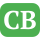CppBuzz.com

# Can you convert this program into Python? Please :)

```#include<iostream>
#include <stdio.h>
#include <stdlib.h>
#include <time.h>
using namespace std;

#define MAXROW 10
#define MAXCOL 10

int main() {

srand(time(NULL));

char choice='n';

do{

int num = 0;
num = rand() % 50; //min no can be 1 - 49
int i, j, k, min, max, myMatrix[MAXROW][MAXCOL];
int row, column, set = 0;
min = max = 0;
int n;

cout<<"\n\nEnter size of Array (2 - 10) : ";
cin>>n;

if(n<2 && n> 10){
cout<<"\n Error. Size of should be 2 to 10";
return -1;
}

//initialize Array
for(int i=0; i<n; i++){
for(int j=0; j<n; j++){
myMatrix[i][j] = num++;
}

}

//print array
for(int i=0; i<n; i++){
for(int j=0; j<n; j++){
int rand1 = rand() % n;
int rand2 = rand() % n;

int ran = myMatrix[rand1][rand2];
myMatrix[rand1][rand2] = myMatrix[i][j];
myMatrix[i][j] =  ran;
}
}

//print array
for(int i=0; i<n; i++){
for(int j=0; j<n; j++){
cout<<" "<<myMatrix[i][j]<<"\t";
}
cout<<"\n";
}

// find the saddle points in the given myMatrix
for (i = 0; i < n; i++) {
row = 0;
min = myMatrix[i];
for (j = 0; j < n; j++) {
if (min > myMatrix[i][j]) {
min = myMatrix[i][j];
column = j;
}
}

//cout<<" \t Min No is " << min;
max = myMatrix[i][column];
for (k = 0; k < n; k++) {
if (max < myMatrix[k][column]) {
max = myMatrix[k][column];
}
}
//cout<<"\t Max no is : " <<max;

// saddle point is - min of a MAXROW and max of the column
if (min == max) {
cout<<"\n Row : "<<i+1;
cout<<"\n Column : "<<column+1;
cout<<"\n Saddle Point : "<< max;
set = 1;
break;
}
}

if(set==0)

cout<<"\n\n Do you want to try one more time ? y/n : ";
cin>>choice;

}while(choice=='y');

cout<<"\n Thank you ^.^";

return 0;
}
```

Posted by TimTim 2018-09-11 19:13:30

`Sure. We will send you solution in 2 days`Admin posted 2018-09-26 21:09:42

`Thanks :) By the way Is the solution free? `TimTim posted 2018-09-26 21:14:19

`Thanks :) By the way Is the solution free? `TimTim posted 2018-09-26 21:14:28

```#this is working code

from random import randint

MaxRow = 10
MaxCol = 10
no = 0

no = randint(1, 50)
i = 0
j = 0
k = 0
minvar  = 0
maxvar = 0
n = 0
row = col = flag = 0

n = input('Enter Size of Array (2 - 10) : ')
n = int(n)

if n < 2 and n > 10:
print('Error. Size should be 5 - 10')

myMatrix = []

for i in range(0,n):
myMatrix.append([])

for i in range(0,n):
for j in range(0,n):
myMatrix[i].append(no)
no = no + 1

#suffle elements

for i in range(0,n):
for j in range(0,n):
rand1 = randint(0, n-1)
rand2 = randint(0, n-1)
temp = myMatrix[rand1][rand2]
myMatrix[rand1][rand2] = myMatrix[i][j]
myMatrix[i][j] =  temp

for i in range(0,n):
for j in range(0,n):
print(myMatrix[i][j] ,end=" ")
print()

for i in range(0,n):
row = 0
minVal = myMatrix[i]
for j in range(0,n):
if minVal > myMatrix[i][j]:
minVal = myMatrix[i][j]
col = j

maxVal = myMatrix[i][col]

for i in range(0,n):
for j in range(0,n):
if maxVal < myMatrix[i][col]:
maxVal = myMatrix[k][col]

if minVal == maxVal:
flag = 1
#print('Row %s' % i+1)
#print('Col %s' % j+1)
print('Number is : %d' % maxVal)

if flag == 0:
print('No Saddle Point Found in Array')```Admin posted 2018-09-29 23:45:42

```# this is with loop

from random import randint

while True:

MaxRow = 10
MaxCol = 10
no = 0

no = randint(1, 50)
i = 0
j = 0
k = 0
minvar  = 0
maxvar = 0
n = 0
row = col = flag = 0

n = input('Enter Size of Array (2 - 10) : ')
n = int(n)

if n < 2 and n > 10:
print('Error. Size should be 5 - 10')

myMatrix = []

for i in range(0,n):
myMatrix.append([])

for i in range(0,n):
for j in range(0,n):
myMatrix[i].append(no)
no = no + 1

#suffle elements

for i in range(0,n):
for j in range(0,n):
rand1 = randint(0, n-1)
rand2 = randint(0, n-1)
temp = myMatrix[rand1][rand2]
myMatrix[rand1][rand2] = myMatrix[i][j]
myMatrix[i][j] =  temp

for i in range(0,n):
for j in range(0,n):
print(myMatrix[i][j] ,end=" ")
print()

for i in range(0,n):
row = 0
minVal = myMatrix[i]
for j in range(0,n):
if minVal > myMatrix[i][j]:
minVal = myMatrix[i][j]
col = j

maxVal = myMatrix[i][col]

for i in range(0,n):
for j in range(0,n):
if maxVal < myMatrix[i][col]:
maxVal = myMatrix[k][col]

if minVal == maxVal:
flag = 1
#print('Row %s' % i+1)
#print('Col %s' % j+1)
print('Number is : %d' % maxVal)

if flag == 0:
print('No Saddle Point Found in Array')

choice = 'n'
choice = input('
Do you want to continue ? y/n : ')
if choice != 'y':
break
```Admin posted 2018-09-30 14:33:04

```it is not printing row and column
```TimTim posted 2018-10-01 06:42:51

```Hi Timtim,

You have to put these two lines, in place of commented #print('Row %s' % i+1) #print('Col %s' % j+1)

print('Row %d' % int (i+1))
print('Col %d' % int (j+1))```Admin posted 2018-10-01 07:36:22

```Hello. I tried this code but it doesn't show the right row and column :) Can you please check?
It always get the number in the corner```TimTim posted 2018-10-03 04:14:22

```#put this code to get correct row and column

if minVal == maxVal:admin posted 2018-10-07 21:53:07